# 运算符

Python语言支持以下类型的运算符:

• 算术运算符
• 比较（关系）运算符
• 赋值运算符
• 逻辑运算符
• 位运算符
• 成员运算符
• 身份运算符
• 三目运算符

## 一、 算术运算符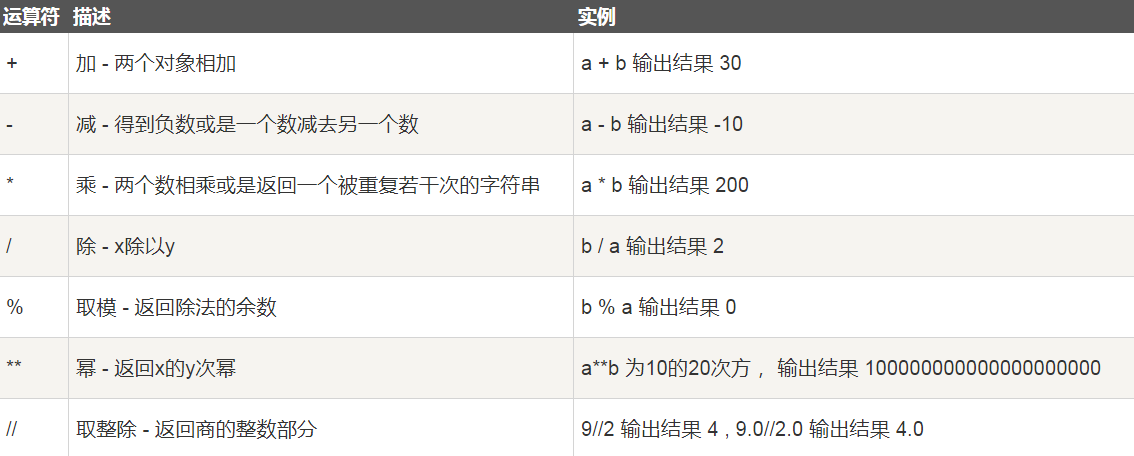Python中，有3种除法，一种除法是`/`

```>>> 10 / 3
3.3333333333333335
```

`/`除法计算结果是浮点数，即使是两个整数恰好整除，结果也是浮点数：

```>>> 9 / 3
3.0
```

```>>> 10 // 3
3
```

Python还提供一个余数运算，可以得到两个整数相除的余数：

```>>> 10 % 3
1
```

```>>> divmod(10,3)
(3, 1)
```

```>>> 0.1+0.1+0.1-0.3
5.551115123125783e-17
```

```>>> from decimal import Decimal
>>> Decimal('0.1')+Decimal('0.1')+Decimal('0.1')-Decimal('0.3')
Decimal('0.0')
>>> Decimal('0.1') / Decimal('0.3')
Decimal('0.3333333333333333333333333333')

>>> from decimal import getcontext
>>> getcontext().prec = 4 #设置全局精度
>>> Decimal('0.1') / Decimal('0.3')
Decimal('0.3333')
```

## 二、 比较运算符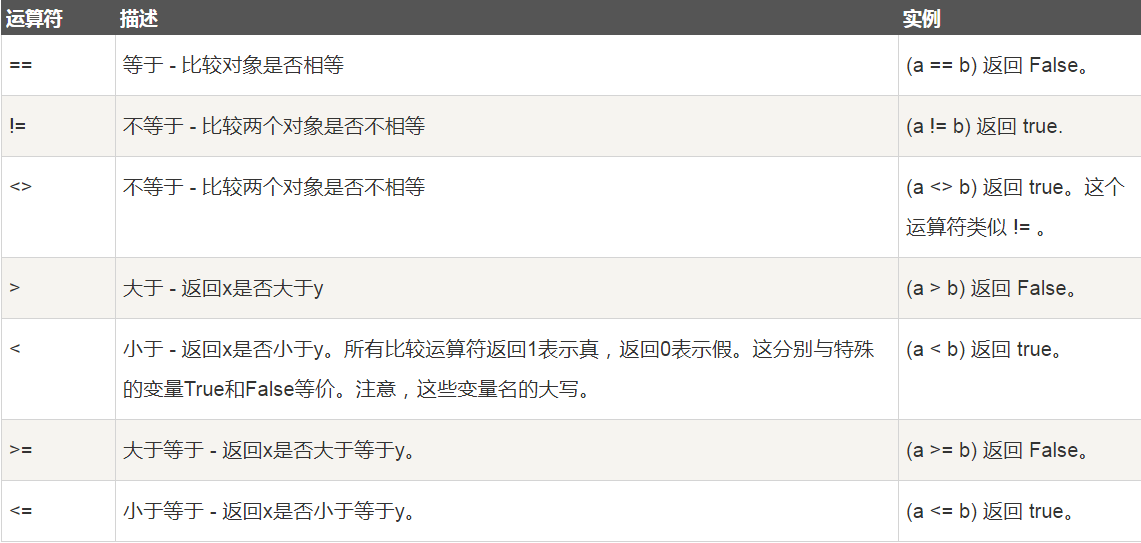1.下面三个选项哪个为True？

• 5+4j > 2-3j
• 'abc' < 'xyz'
• (3, 2) < ('a', 'b')

2.请分别说出下面各项的值：

• True == 1
• False == 0
• 3>2>1
• 3>2>2
• (3>2)>1
• (3>2)>2

`3>2>1 等于 (3>2) and (2>1)`

## 三、 赋值运算符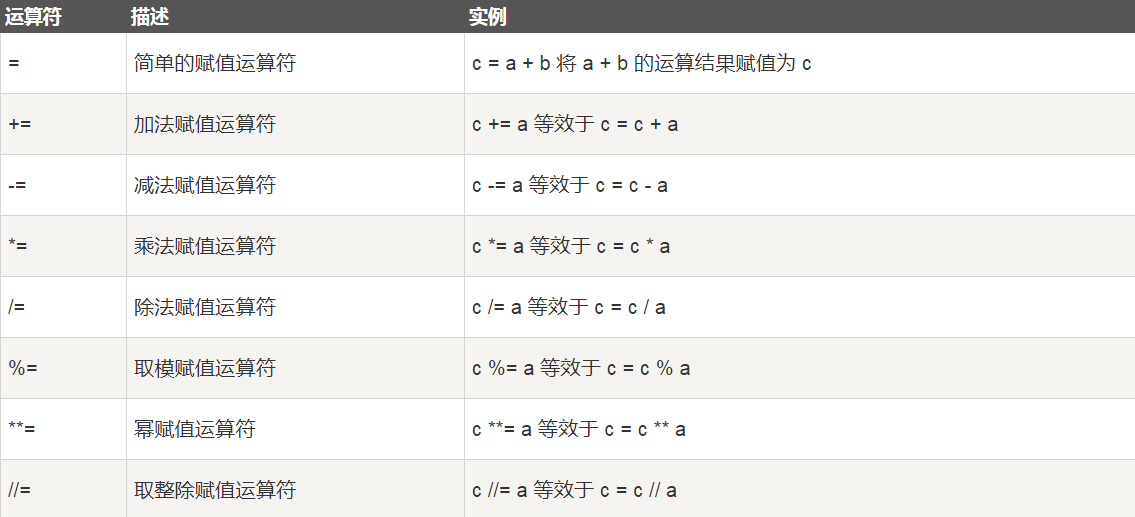## 四、 位运算符

```a = 0011 1100

b = 0000 1101

-----------------

a&b = 0000 1100

a|b = 0011 1101

a^b = 0011 0001

~a  = 1100 0011
```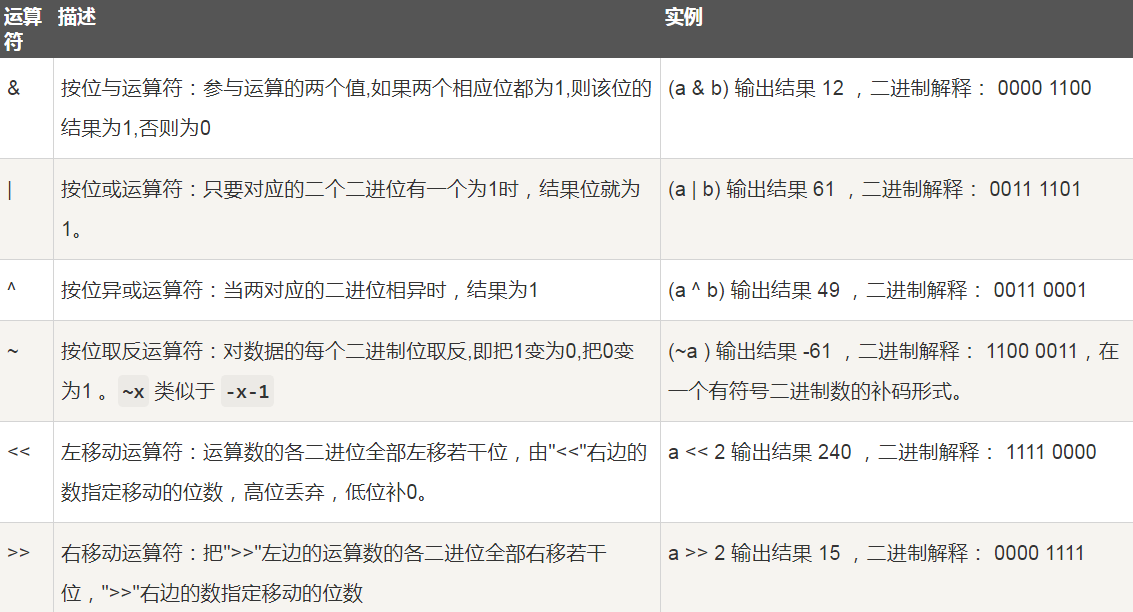## 五、 逻辑运算符

Python语言支持逻辑运算符，但是没有其它语言中的`&&``||`语法，取而代之的是更加人性化的英文单词`and or not`（全部都是小写字母）。以下假设变量 a 为 10, b为 20: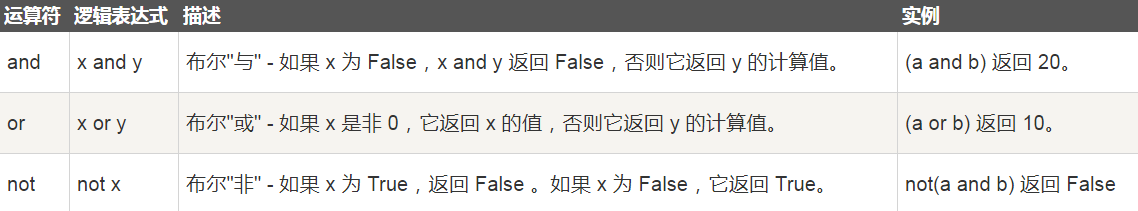## 六、 成员运算符

`in 与 not in`是Python独有的运算符（全部都是小写字母），用于判断对象是否某个集合的元素之一，非常好用，并且运行速度很快。返回的结果是布尔值类型的True或者False。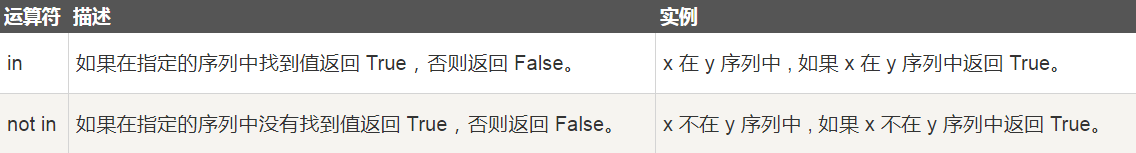```list1 = [1, 2, 3, 4, 5]
a = 1
flag = False

for i in list1:
if i == a:
flag = True
break

if flag:
print("a是list1的元素之一")
else:
print("a不是list1的元素")
```

```list1 = [1, 2, 3, 4, 5]
a = 1
if a in list1:
print("a是list1的元素之一")
else:
print("a不是list1的元素")
```

## 七、 身份运算符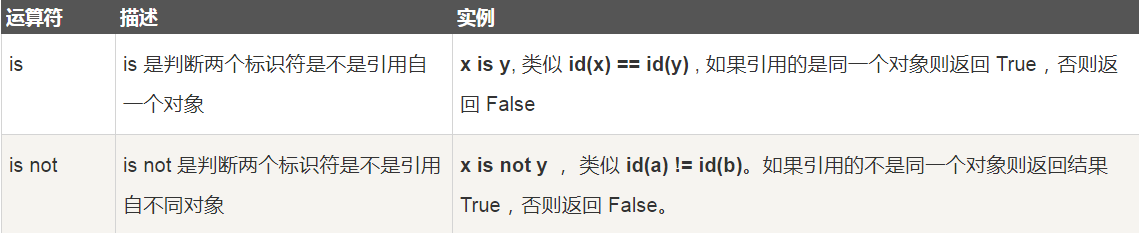is用于判断两个变量的引用是否为同一个对象，而==用于判断变量引用的对象的值是否相等！

```>>> a = [1, 2, 3]
>>> b = a
>>> b is a
True
>>> b == a
True
>>> b = a[:]
>>> b is a
False
>>> b == a
True
```

```>>> a = 1
>>> b = 2
>>> id(a)
1383969856
>>> id(b)
1383969888
>>> c = 2
>>> id(c)
1383969888
```

```>>> 3.0 is 9/3
True
>>> id(3.0)
2434753652496
>>> id(9/3)
2434747931216
>>> 3.0 is 9/3
True
>>> a = 3.0
>>> id(a)
2434747931216
>>> b=9/3
>>> id(b)
2434753652496
>>> a is b
False
>>> 1333 is 3999/3
False
>>> 1333.0 is 3999/3
True
>>> id(1333.0)
2434751213232
>>> id(3999/3)
2434751213328
>>> 133333.0 is 399999/3
True
>>>
```

## 八、 三目运算符（三元表达式）

python中的三目运算符不像其他语言一般的表示方法：`判定条件?为真时的结果:为假时的结果`

## 九、 运算符优先级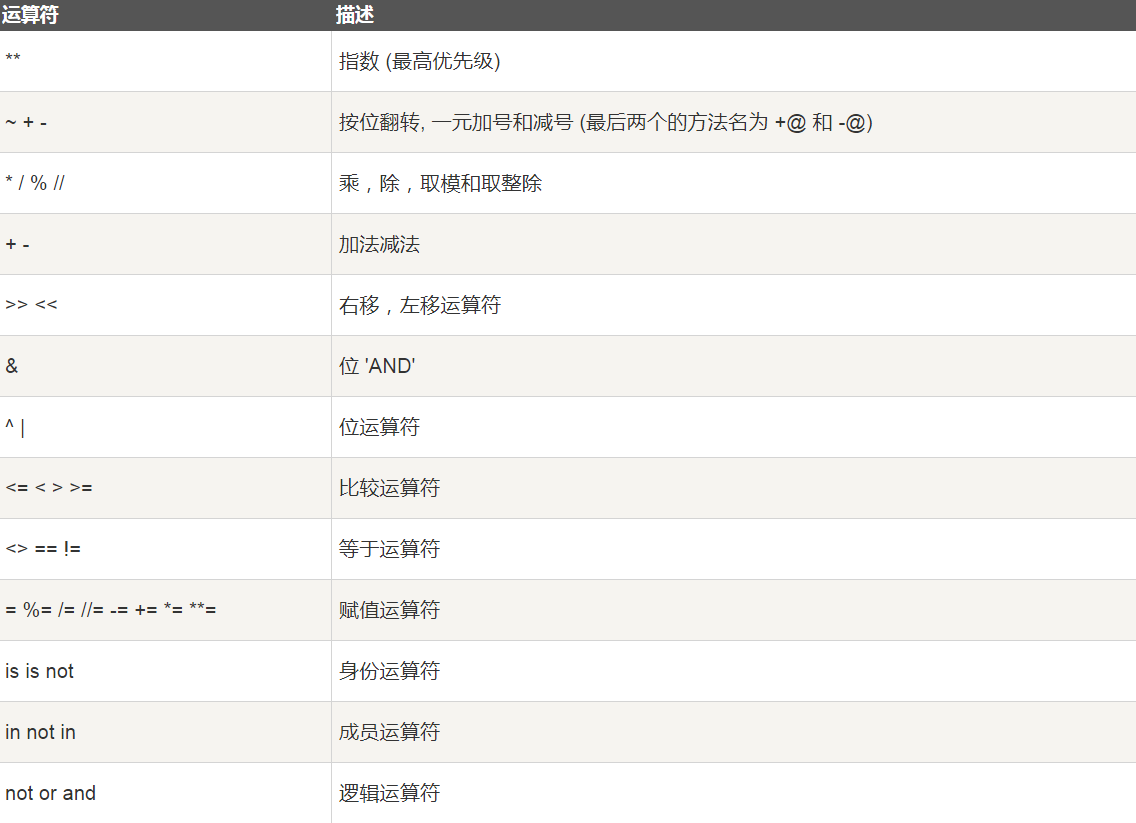#### 评论总数： 2